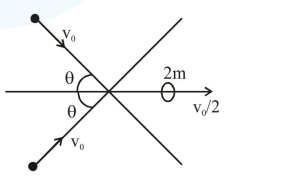# Two bodies of the same mass are moving with the same speed, but in different directions in a plane.

Question:

Two bodies of the same mass are moving with the same speed, but in different directions in a plane. They have a completely inelastic collision and move together thereafter with a final speed which is half of their initial speed. The angle between the initial velocities of the two bodies (in degree) is.

Solution:Momentum conservation along $x$

$2 \mathrm{mv}_{0} \cos \theta=2 \mathrm{~m} \frac{\mathrm{v}_{0}}{2}$

$\cos \theta=\frac{1}{2}$

$\theta=60$

Angle is $2 \theta=120$

Ans. $120.00$